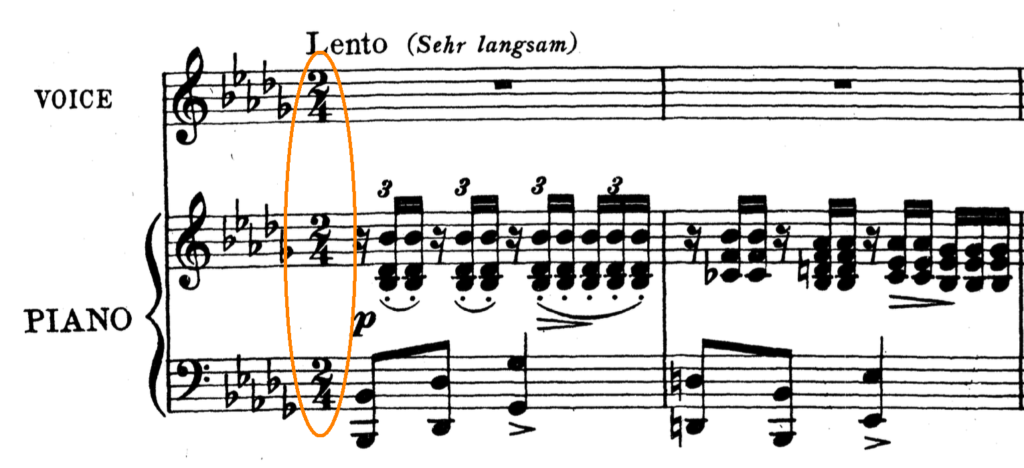# Time Signatures & Meters – Everything you need to know

Before we get into actual time signatures, we have to talk a little bit about meter. After all, time signatures represent meter in written music.

Meter is the division of beats into equal groups. We’ve already talked about this briefly in the How to Read Music course (part 2) but here’s a quick demonstration.

This is a pulse made up of 6 quarter notes: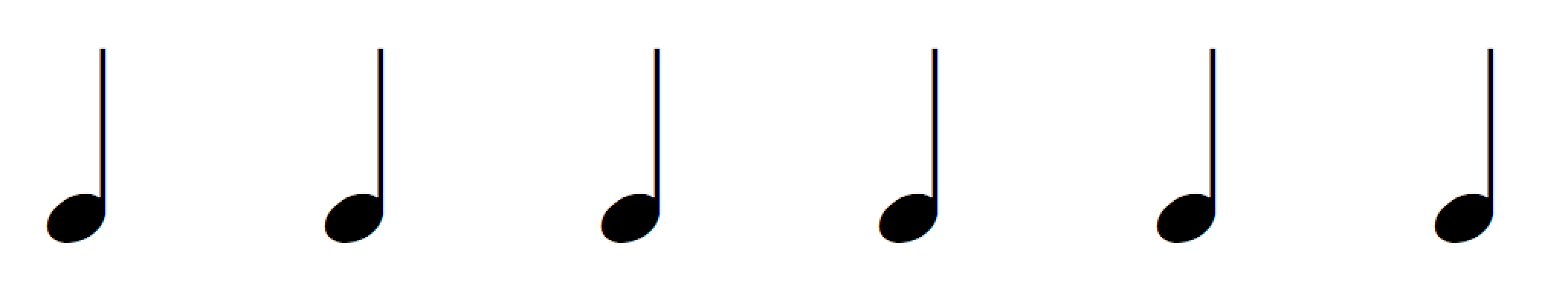Although it’s quite simple, it can be played in different ways. Let’s try one possibility where the 6 quarter notes are divided into 2 groups of 3 quarter notes: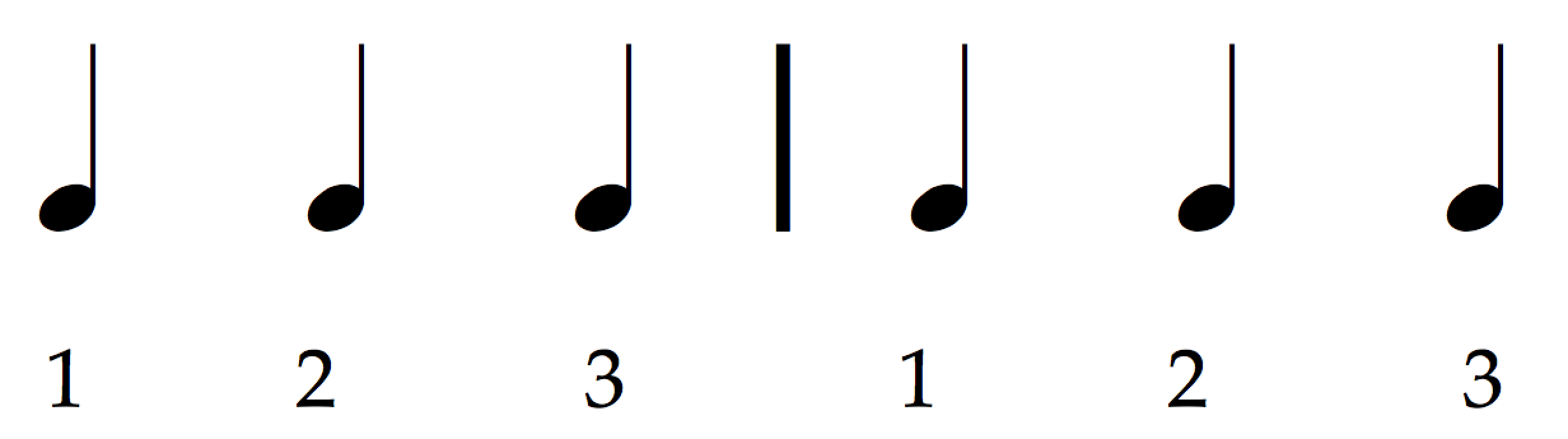Listen to example:

Now let’s try a different one. This time the pulse is divided into 3 groups of 2 quarter notes: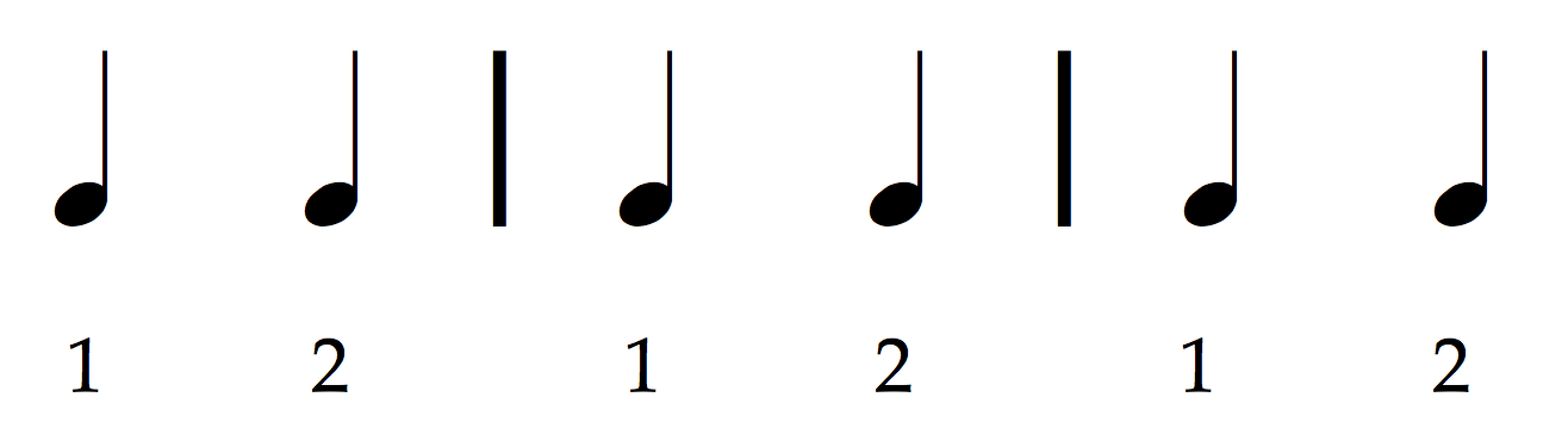Listen to example:

I’m sure we can agree that these pulses are very different in their musical effect.

Now the important question is:

What's in this Lesson

## How did we distinguish that these two are different at all?

The answer is in the accents. Just like in everyday language where some words are more stressed than others, in music some beats are stronger than others (or we can say, some beats are accented).

In the first example above, the 6 quarter notes are divided into 2 groups of 3 beats each. The first beat of every group is stronger than the other two; or we can say that it’s accented. And so we perceive the meter as being ‘in three’ meaning that the pulse has an ongoing ‘one, two, three, one, two, three, etc.’ effect. Another term for ‘in three’ is to say that the meter is ‘triple’.

In the second example, the strong beat appeared every 2 beats and so we perceive the meter to be ‘in two’ – its effect is of a recurring ‘one, two, one, two, etc.’, where the ‘one’ is strong (accented) and the ‘two’ is weaker. Another term for ‘in two’ is ‘duple meter’.

These cycles of strong and weak beats are better known in music as meters. They can be in any amount of beats but the most common are the ones in two (or duple meters), in three (or triple meters) and four (or quadruple meters).

In musical notation these groups of beats are organized into measures (also known as bars) and separated by bar lines.## Time Signature

Now we can get to Time Signatures. As you’ve probably seen in sheet music, a time signature is a symbol that is made up of two numbers – one sitting on top of the other. Time signatures are used to indicate the meter quickly and clearly at the beginning of a piece of music.

In sheet music you’ll find the time signature at the beginning of the music. Here’s an example from a song by Schubert.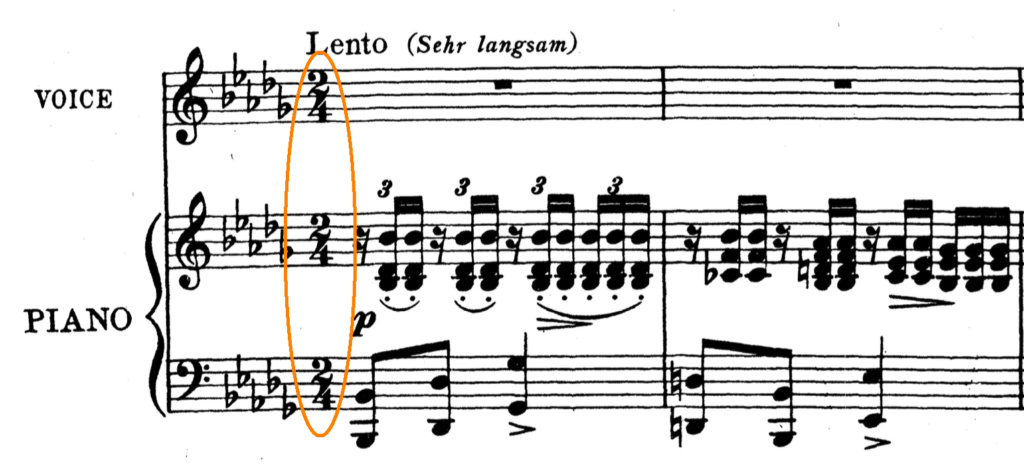So in this Schubert song, the time signature is two-four: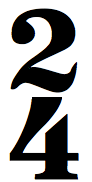### So what do these numbers mean?

The number on top tells us the amount of beats per measure. It tells us whether the meter is in two, three, four or more. The time signature shows that the meter is in two; there are two beats in every measure.

The bottom number tells us what those beats are worth. It shows us whether the beats are eighth notes, quarter notes, half notes, or another note value.

This bottom number is relative to the whole note. The easiest way to work out what the beats are worth is to divide the whole note by that bottom number. For example, if the bottom number is a 2, we work out ‘whole note divided by 2’ which gives us two half notes. So when the bottom number of a time signature is a 2, the beats are half note beats.

What about a four? The whole note divided into 4 gives us 4 quarter notes. So a time signature with 4 as the bottom number represents a quarter note pulse.

 Bottom Number Resulting Beat 1 whole note beat 2 half note beat 4 quarter note beat 8 eighth note beat 16 sixteenth note beat

and so on..

In the example above, the bottom number is a 4 so each beat is a quarter note. So the time signature two-four indicates that the meter is 2 quarter beats per measure. This is a duple meter, which means that it’s made of two recurring beats – the first beat is the strongest, the second being the weaker.

Here’s a composition in two-four meter. Listen carefully to the ‘one – two – one – two’ feel to it.

Listen to example: Beethoven: Turkish March, Op. 113

Let’s look at another example. This is the time signature three-eight: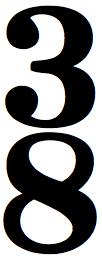Since the top number is a three, then we know that this meter consists of 3 beats per measure. It is a triple meter. Now we need to figure out what kind of beats they are. Since the bottom number is an eight, then they are eighth notes.

Three-eight is a triple meter of 3 eighth notes per measure.

Here’s a composition in three-eight meter. Try to notice the one – two – three – one – two – three feeling.

Listen to example: Haydn – Allegro from Sonata no. 8

And what about the time signature two-two: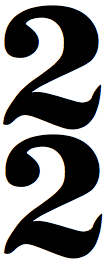The number on top tells us that there are two beats per measure. What kind of beats? The bottom number, also a two, indicates that they are half note beats. So this meter is a duple meter and it consists of two half note beats per measure.

Here’s a piece of music in two-two meter. Just like two-four, listen to the ‘one – two – one – two’ effect.

Listen to example: Mozart: Symphony no. 40 in G minor, 1st movement

Now try it out for yourself. Before we move on, define this time signature: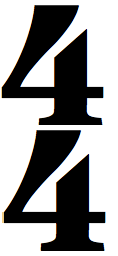– – – – – – – – – – – – – Read ahead when you’re ready – – – – – – – – – – – –  –

The bottom number tells us that the beat is a quarter note beat. How many quarter note beats per measure? From the top number we know that it’s four. So this is a quadruple meter that consists of four quarter note beats per measure.

## Strong & Weak Beats

Before we move on, keep in mind that meters are made up of a fixed layout of strong and weak beats. However, don’t let the words ‘strong’ and ‘weak’ mislead you. They are terms that allow us to explain this particular aspect of rhythm. There is nothing inherently better with strong beats or worse with weak beats. They are both equally important. It is with these two together that meter works.

In duple meters, the first beat is the strongest while the second is weak. The example here is in two-four meter but the same applies to any duple meter such as two-eight or two-two. In the following diagrams, S stands for strong and w stands for weak. An uppercase W stands for weakest.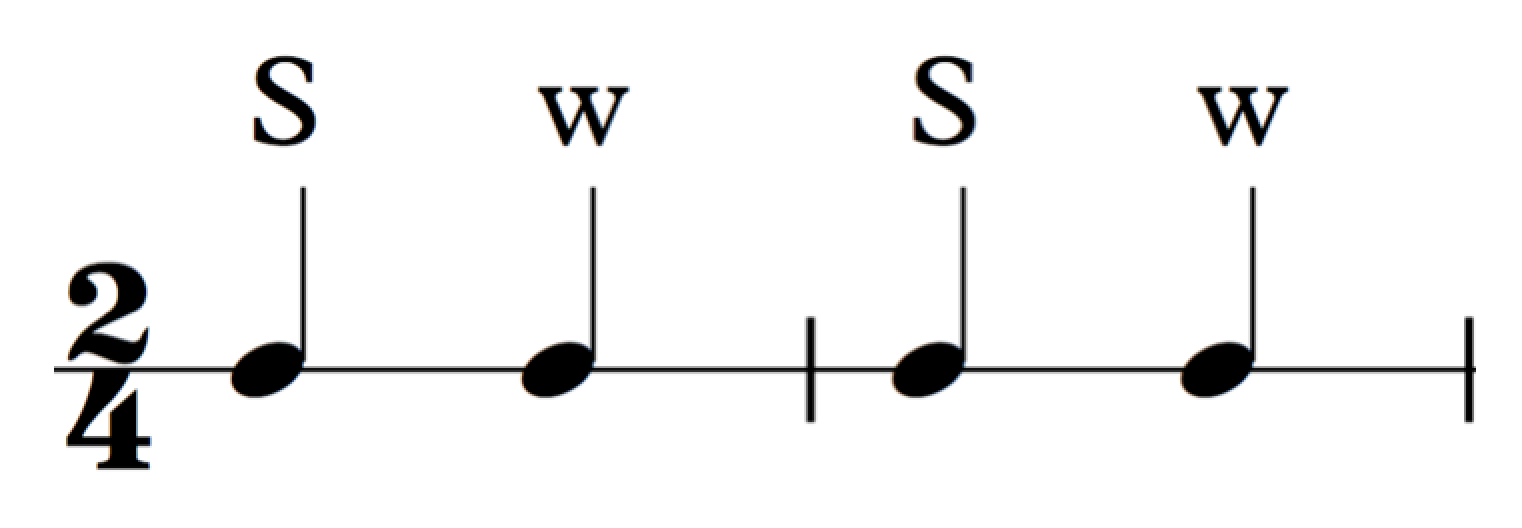Listen to example:

In triple meters, the first beat is the strong and it is followed by two weak beats.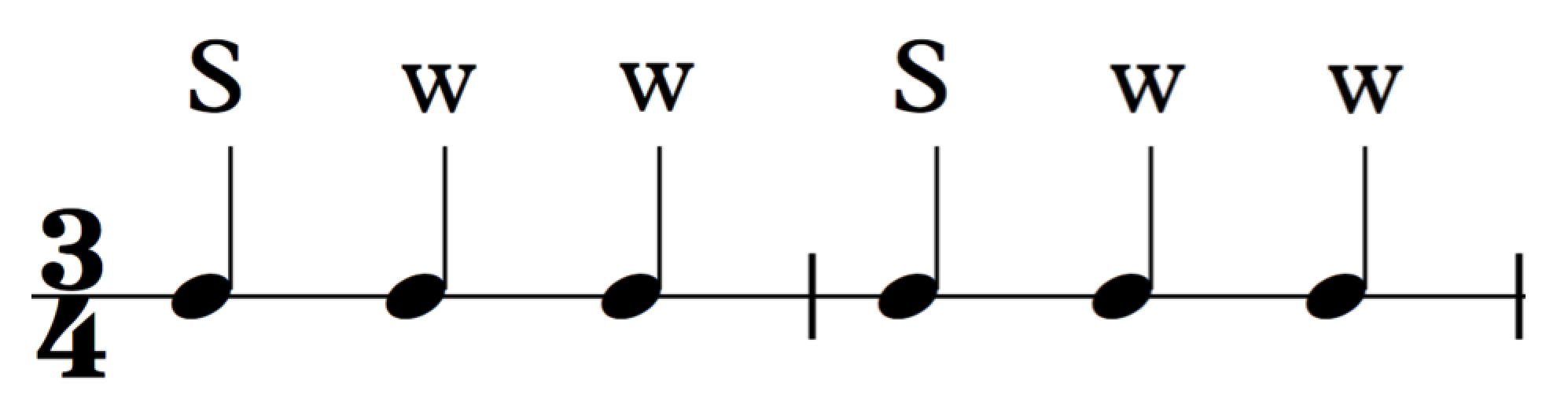Listen to example:

In quadruple meters, the first and third beat are strong and the second and fourth are weak. The first beat, however, is stronger than the third so the four beats are: Strongest, weak, strong, weak.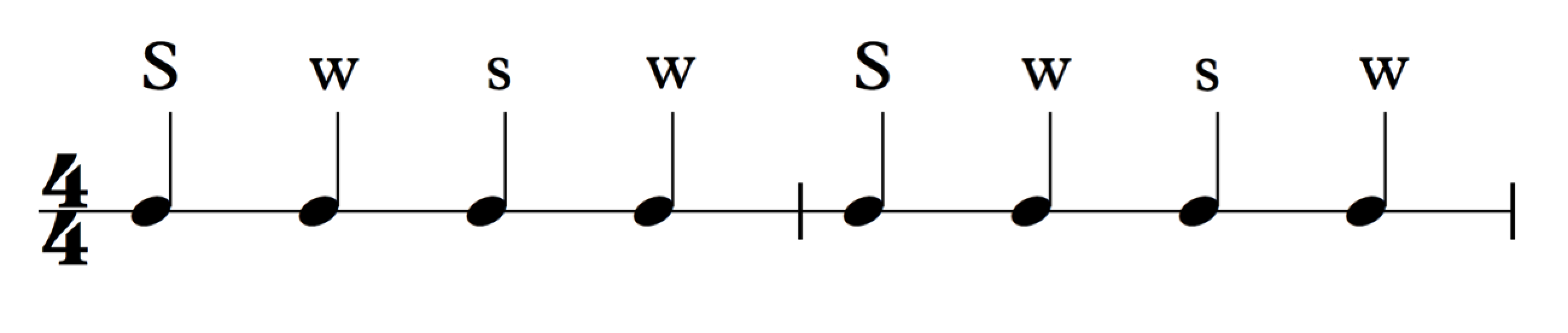Listen to example:

It’s important to note that these strong and weak beats are not forced by musicians – they occur naturally within melodies, chord progressions, accompaniments and so on because music is naturally made up of patterns.

Also, the impression of strong and weak beats of a meter is often altered according to the flow of the music. A strong beat can be felt earlier or later than expected. In fact, this is a common way of giving the music a sense of variety. But it’s important to understand this theoretical outline of meters in order to understand any diversions from it.

## The Eighth Note Beat

We’ve learned already that when the pulse consists of quarter note beats, the bottom number of the time signature is 4. When the pulse consists of eighth note beats, the bottom number of the time signature is 8.

As always, the top number of the time signature shows us the amount of beats per measure. The bottom number shows us what each of those beats are worth – in this case, eighth notes. So with the time signature below, we get 2 eighth note beats per measure. Just like two-four, it is made up of two beats per measure.Similarly, this is 3 eighth note beats per measure: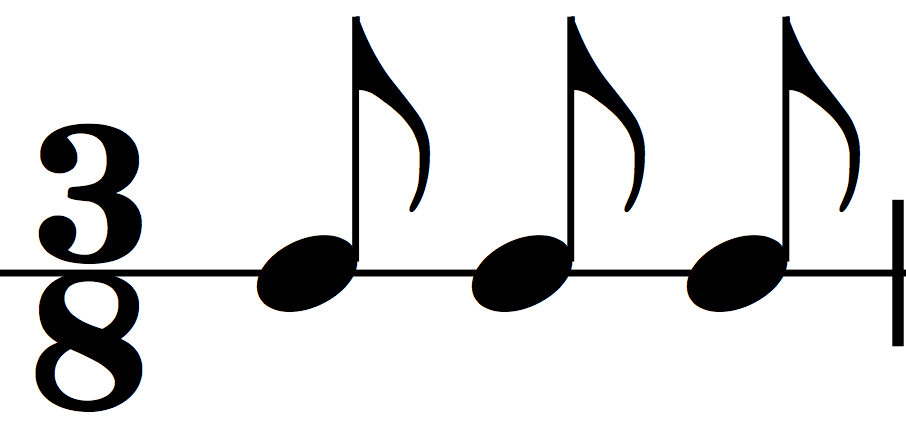And this is 4 eighth note beats per measure: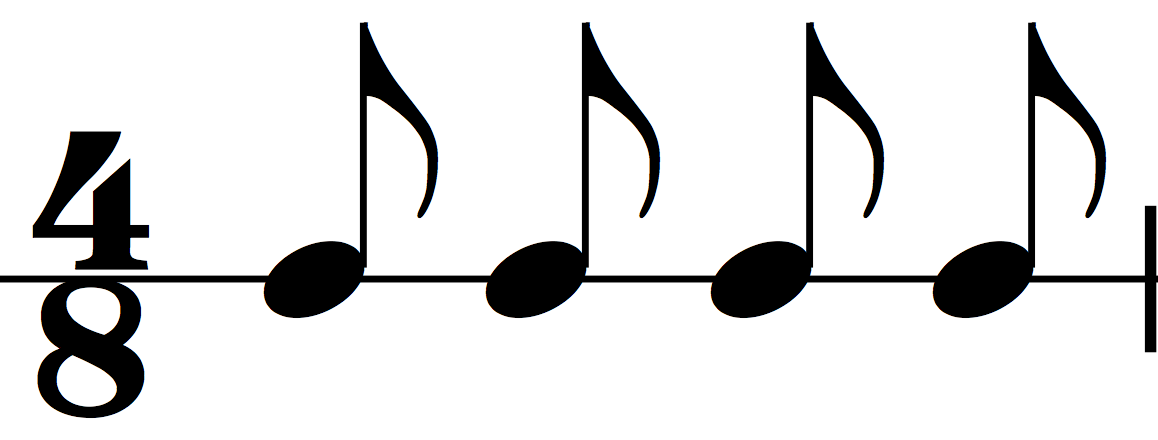The table below shows how the note values relate to different kinds of beats. The quickest way of calculating these relationships is to keep in mind which note value is assigned the value of 1.

The sixteenth note, for example, is one fourth of a beat if the beat is a quarter note. In other words, when the quarter note equals ‘one’, the sixteenth note equals ‘one-fourth’. But when the beat is an eighth note, then that same sixteenth note becomes half a beat. That is, if the eighth note equals ‘one’, the sixteenth note equals ‘one-half’.## The Half Note Beat

Now let’s look at meters where the pulse consists of half note beats. In the time signature, half note beats are indicated with a number 2 at the bottom.

For example, this is three-two meter.

The bottom number is 2 so the meter consists of half note beats. How many half note beats per measure? According to the number on top: 3. So time signature is ‘3 half note beats per measure’: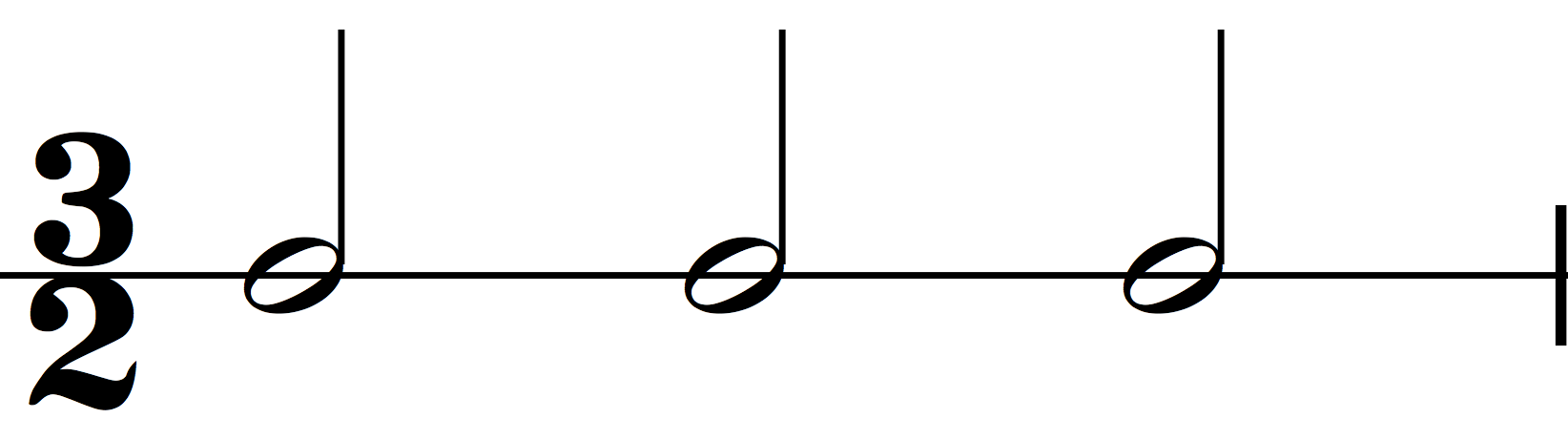Similarly, 2 half note beats per measure: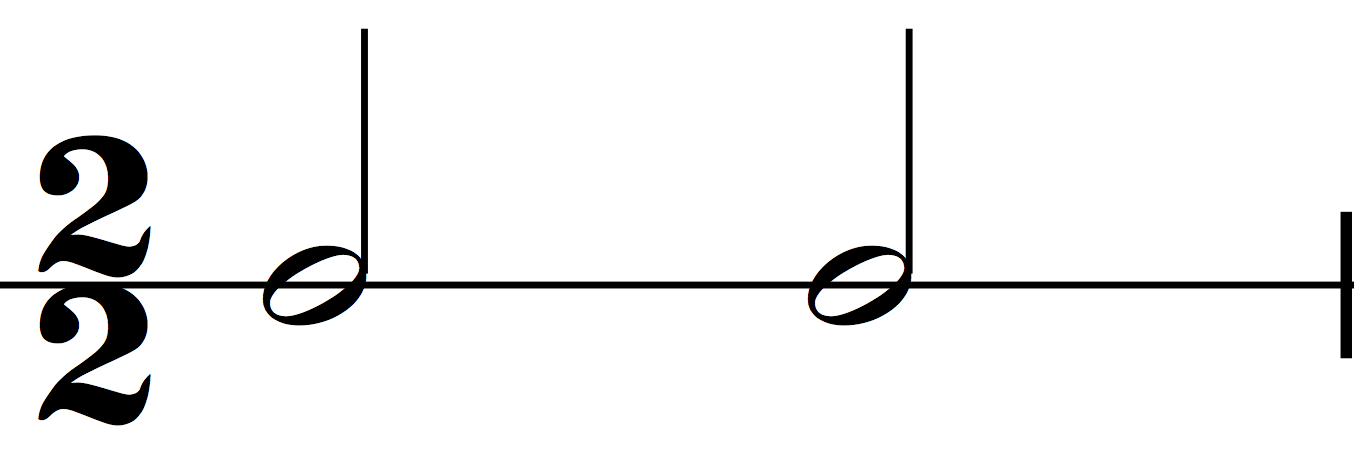And 4 half note beats per measure: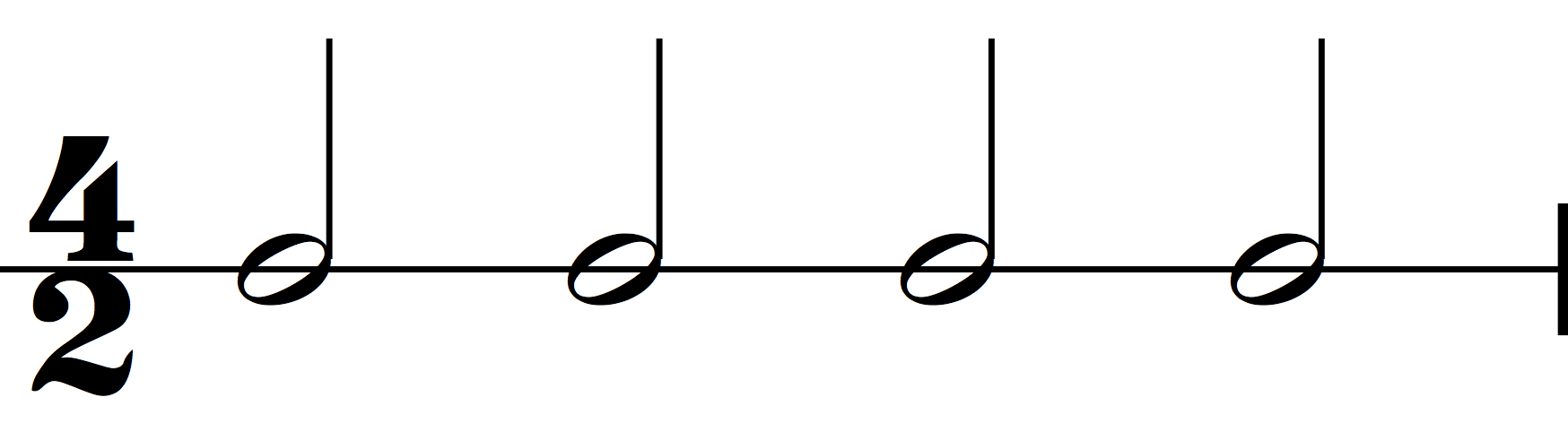Just as before, our note durations acquire a different value depending on the beat. So if the quarter note equals ‘one beat’, then it takes two eighth notes to fill one beat. But if the half note equals ‘one beat’, then it takes four eighth notes to fill one beat.Apart from duple, triple and quadruple, we’ll also categorize meters as either simple or compound.

## Simple Meters

Simple time signatures are those that we’ve covered so far. What makes them simple is that each beat of the meter is naturally divisible by 2 (whereas we’ll see that compound meters are naturally divisible by 3). For example, two-four is a simple meter because every quarter note beat is naturally divisible by 2:So the time signature two-four is a simple duple meter.

Another simple duple meter is two-two. It is duple because it is made up of 2 beats per measure and it is simple because each of those beats is naturally divisible by 2.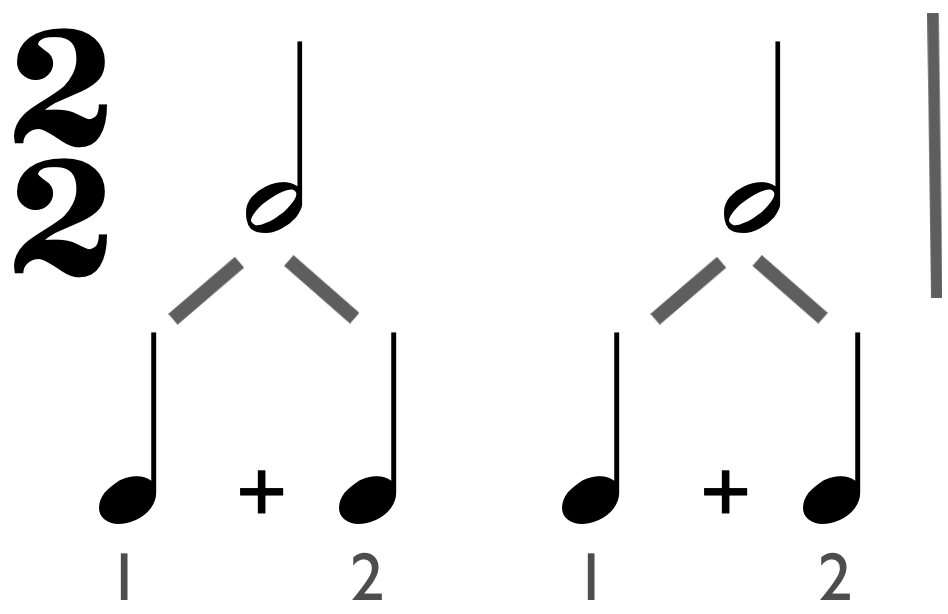## Compound Meter

For every simple meter, there is a compound meter counterpart. While simple meters are those whose beats are naturally divisible by 2, every beat in a compound meter is naturally divisible by 3.

This is how it works: let’s take the simple duple meter two-four. As we’ve seen earlier, it’s made up of 2 quarter note pulses per measure and each of those is naturally divisible into two eighth notes.But if the meter was made up of 2 dotted quarter notes instead: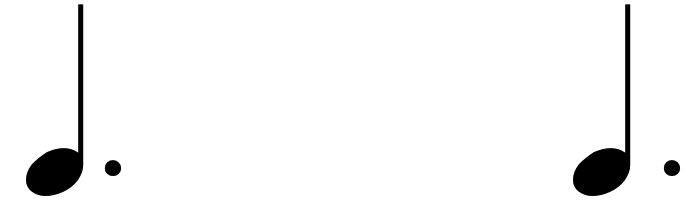Each of these 2 pulses are now naturally divisible into 3 equal parts: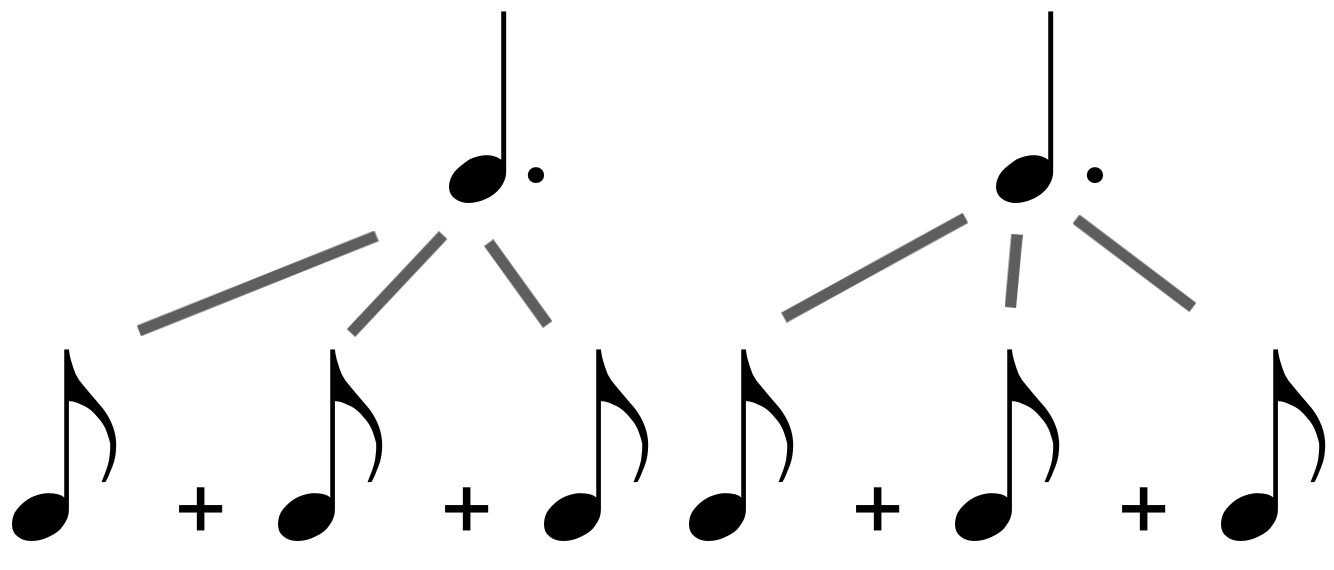In fact, the time signature for a meter made up of 2 dotted quarter notes is six-eight – 6 eighth note beats per measure.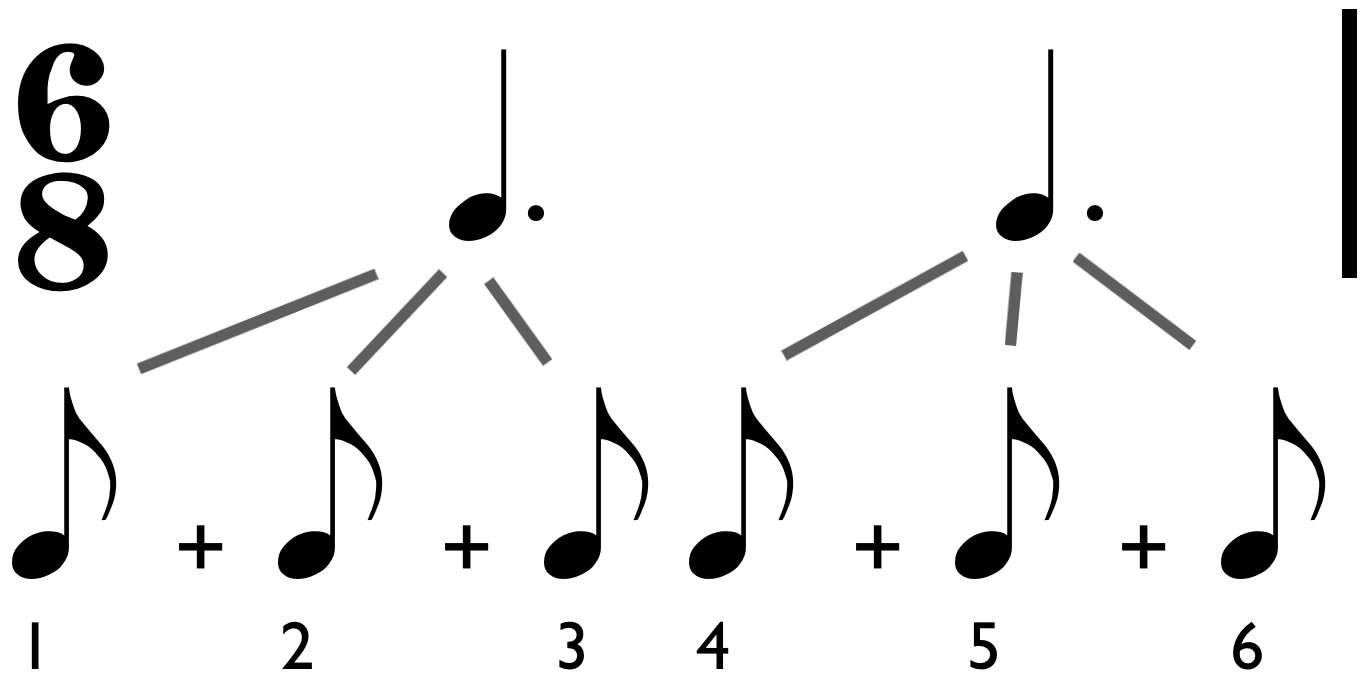Keep in mind, however, that the main pulses are still the two dotted quarter notes (and out of which the first is the strongest). To distinguish between the main beats and their smaller parts, we can use the word ‘subdivision‘:This means that this meter is duple. Since each of the 2 beats is naturally divisible by 3, it is compound. So the time signature six-eight is a Compound Duple Meter.

Let’s look at another example. This is a simple triple meter made up of 3 eighth notes per measure.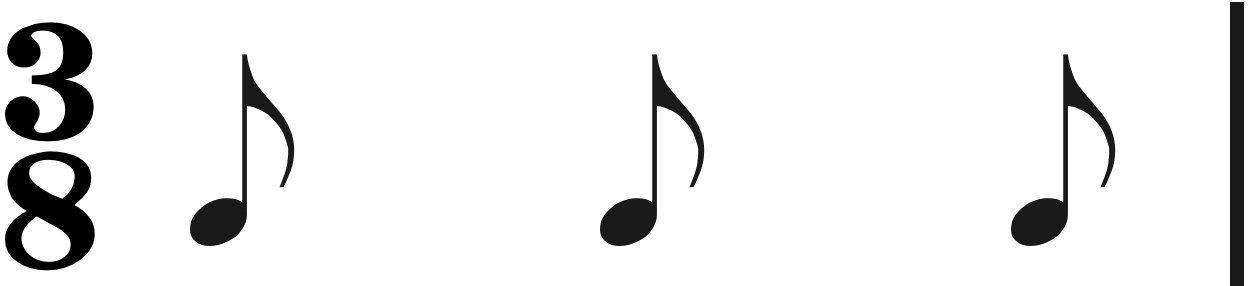Its compound triple counterpart must consist of 3 dotted eighth notes per measure and each of the 3 dotted eighth notes are divisible by 3. And so this is a compound triple meter:Adding those divisions up (the sixteenth notes) we learn that the time signature of this meter is nine-sixteen: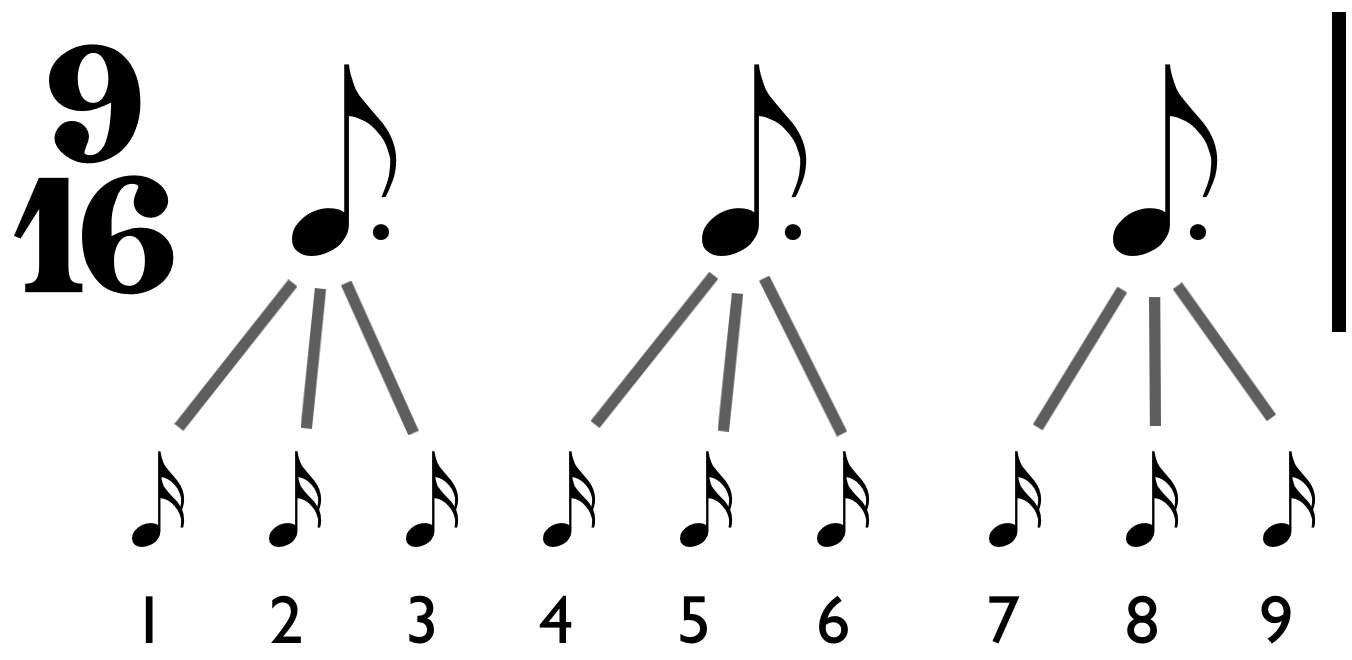Finally, we’ll discuss an example of a compound quadruple meter. This measure is filled with 4 dotted half notes. What is the time signature?Let’s get to the answer step by step. Every dotted half note can be broken down into 3 quarter notes: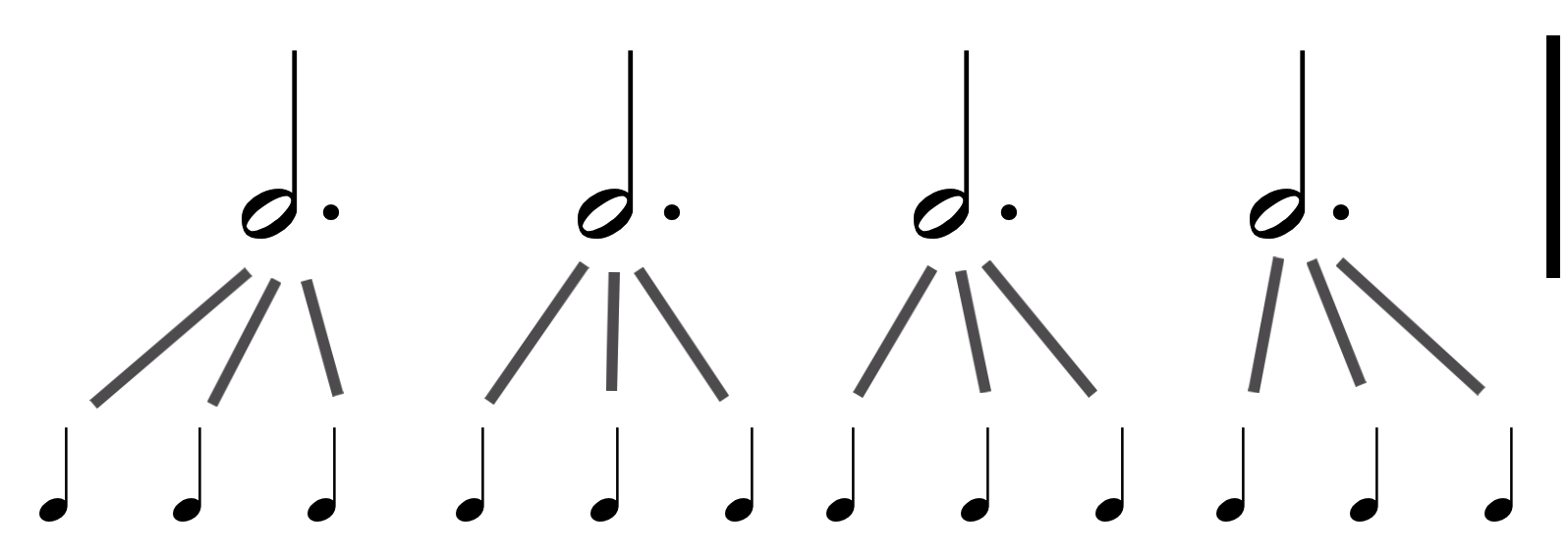The measure is now filled with 12 quarter notes. The top number of the time signature will therefore be 12. The bottom number must represent the quarter note subdivision and from our experience with simple meters we know that this is 4.

So the time signature of this compound quadruple meter is twelve-four. The pulse is in 4 and each one is divisible into 3 quarter notes: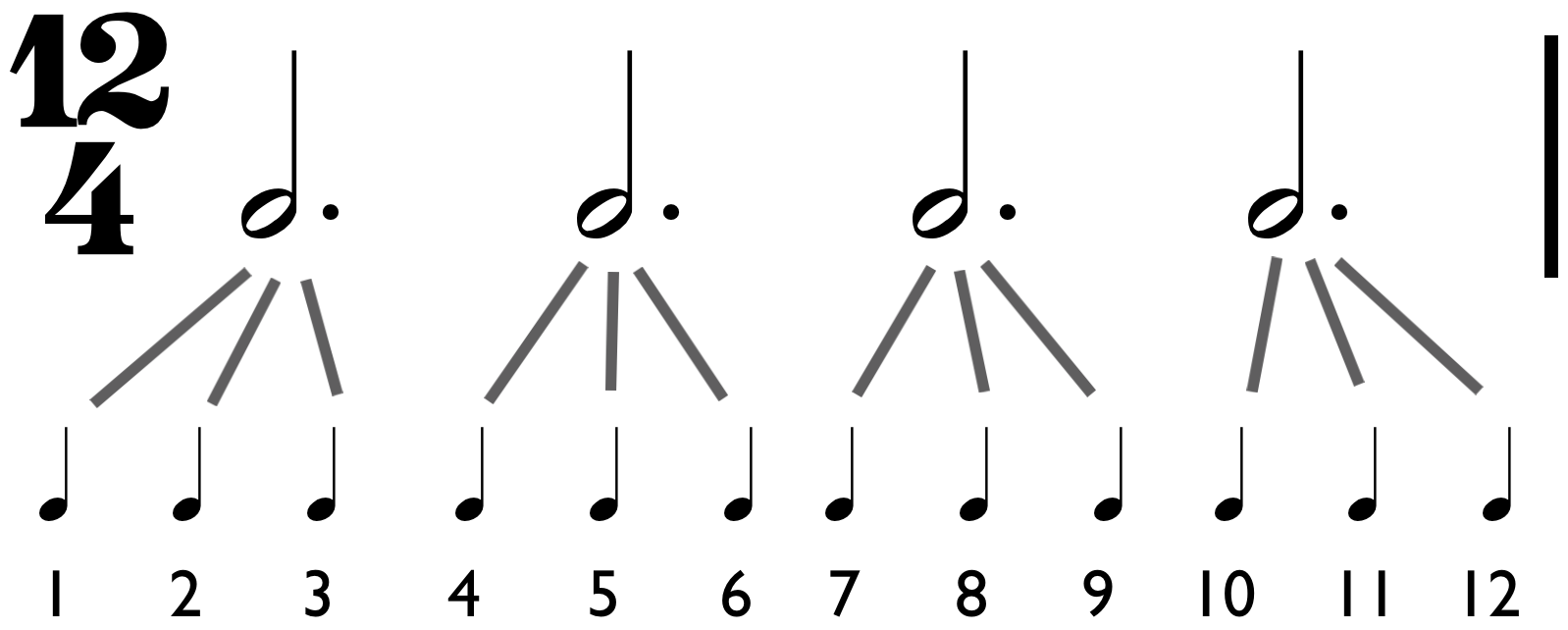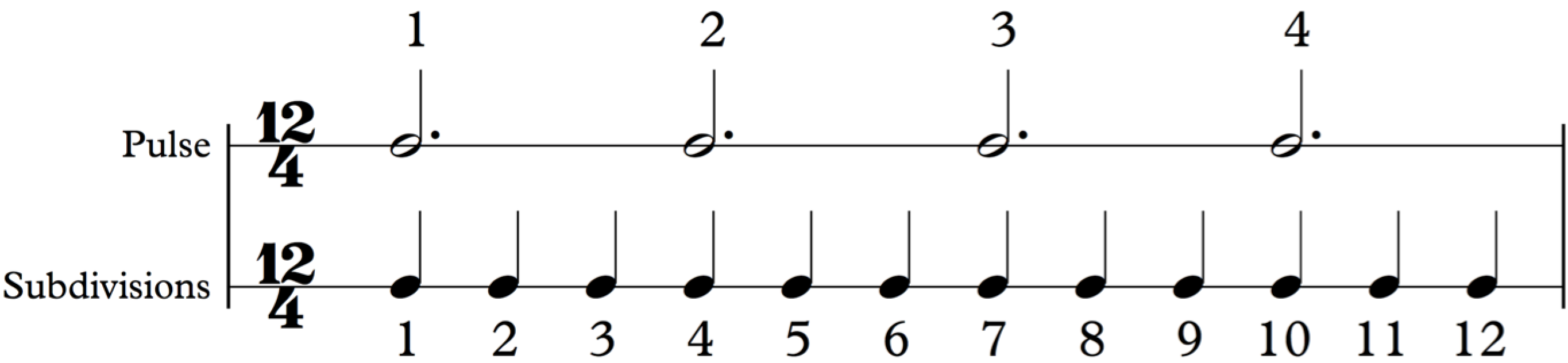So we learned that meters are defined in two ways:

• by the number of beats: duple, triple or quadruple
• and by the natural subdivision of those beats: simple or compound

So that’s all about time signature! Exercises are coming soon 🙂 I’d love to hear your comments or questions so post them below!